Get our FREE VBA eBook of the 30 most useful Excel VBA macros.

Automate Excel so that you can save time and stop doing the jobs a trained monkey could do.

# How to display fractions in ExcelWhen using Excel, we often display numbers as decimals.  However, that is not necessarily the best way for users to read the information; fractions can be a better option.  We could enter fractions as text, but then we can’t use them in calculations.  So, in this post, we’ll look at the best way to use fractions in Excel.

Contents

Let’s start with a brief recap about fractions as it will help us understand what Excel is trying to do.

Fractions are a way of presenting a value as the number of sections of a whole.  For example, 3/4 is a fraction where a whole is 4 parts, and our value is declared as being 3 of those parts.

There are different types of fractions:

• Proper fractions – where the number at the top (the numerator) is less than the number at the bottom (the denominator).  For example, 3/4 is a proper fraction; it represents a number equivalent to 3 parts out of a whole, where a whole is divided into 4 equal sections.
• Improper fractions – where the number at the top (the numerator) is bigger than or equal to the number at the bottom (the denominator).  For example, 5/4 is an improper fraction; it represents a number equivalent to 5 parts of a whole, where a whole is divided into 4
• Mixed fractions – where a number is presented before a fraction.  For example, 1 1/4 is a mixed fraction representing 1 whole, plus 1 part of a whole, where a whole is divided into 4.

## Displaying fractions in a cell

To display a fraction in a cell, we use number formatting.

Number formatting is a way of displaying a value in a specific format, without changing the value itself.  In simple terms, changing the number of decimal places is a form of number formatting.  Look at the screenshot below:Why isn’t the value in cell B4 equal to 4? Surely 2+2=4?  The numbers in B2 and B3 are actually 1.5 but shown with zero decimal places, so they have been rounded up to 2.  The numbers aren’t incorrect; they are just formatted differently.

### Apply number formatting

To apply number formatting, select the cells to be formatted, click Home -> Number -> Dialog Launcher (button) (or press Ctrl + 1) to open the Format Cells window.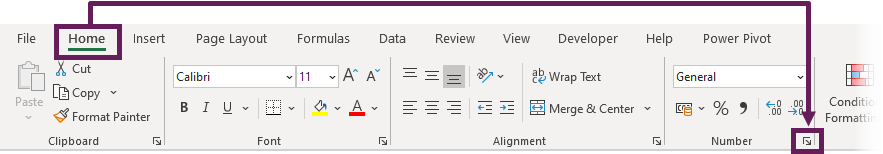From here, we can select the Fraction category and choose the format we want.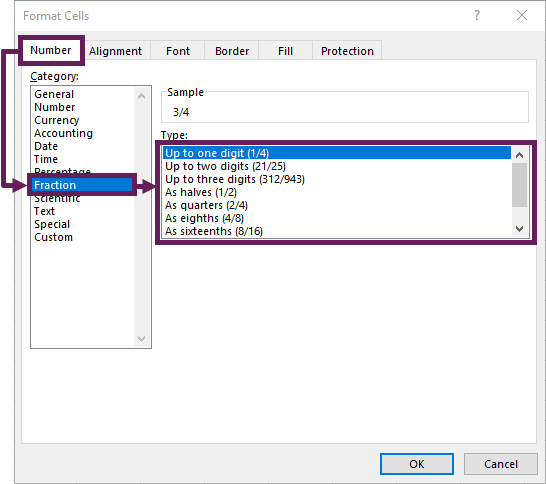The number will now be formatted as a fraction.As you can see from the screenshot above, the number 0.75 is presented as 3/4.

NOTES:

• If the exact fraction cannot be displayed, it will be rounded to the nearest result.
• Fractions in Excel will automatically be converted to the lowest denominator.  For example, a fraction of 4/8 will be converted to 1/2.

### Automatically apply number formatting through data entry

There is a useful little hack for entering fractions faster.  If we enter a mixed fraction into a cell, Excel understands that we want to display a fraction and automatically changes the number format for us.

For example, entering 0 3/4 will automatically convert the format of the cell to a fraction.NOTES:
If we enter a fraction without a leading digit, Excel thinks we want a date or text.  Therefore, it’s important to enter a mixed fraction to trigger the automatic cell formatting.

### Fix the denominator

As mentioned earlier, Excel will automatically reduce fractions to have the lowest denominator.  However, sometimes, we want to fix the denominator.  In this case, we can create a custom number format:

Select the cells to be formatted and click Home -> Number -> Dialog Launcher (button) (or press Ctrl + 1) to open the Format Cells window.

In the custom section, I have entered # ?/16 in the type box, which will create a mixed fraction where the denominator is always fixed as 16.### Enter improper fractions in Excel

To enter improper fractions, we can again use a custom number format.  By entering ??/?? (without a hash at the start), the value will only show a fraction (i.e., it will not display a mixed fraction).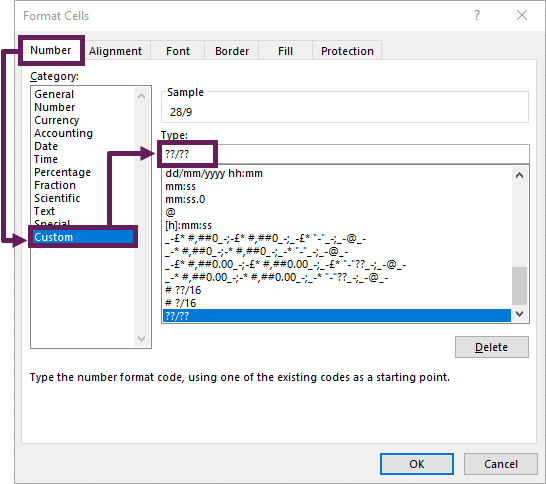We can now mix fixed denominators and improper fractions.  For example, ???/100 will display 120/100 if we enter 1.2 into a cell.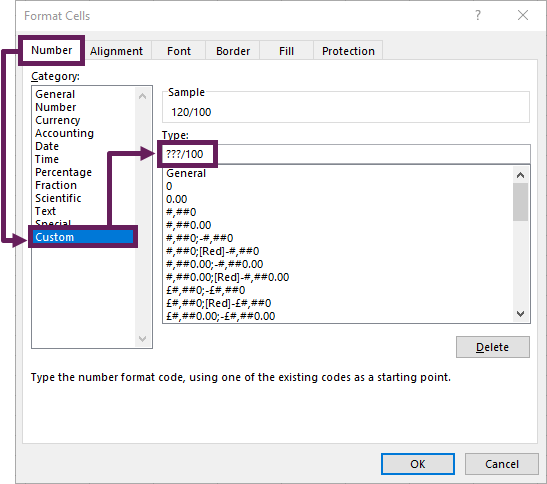## Enter fractions into a text string

Sometimes we want to display the fraction into a text string, rather than just in a cell.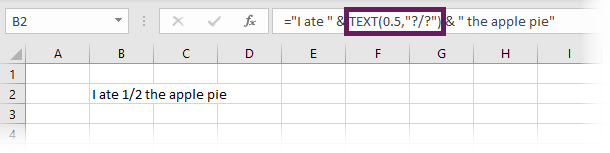The example above is created by using the TEXT function.  In cell B2 the formula is:

`="I ate " & TEXT(0.5,"?/?") & " the apple pie"`

The TEXT function’s first argument is the value; the second argument is the number format to apply.  From this, we can create a fraction by using the same format code as we used in the customer number format.

## Conclusion

In this post, we’ve seen how to use fractions in Excel.  There are three types of fractions. By using the right custom number format, we can decide which of the 3 we will display.  But using a number format, rather than text, we can still use calculations on those numbers.Get our FREE VBA eBook of the 30 most useful Excel VBA macros.

Automate Excel so that you can save time and stop doing the jobs a trained monkey could do.

Don’t forget:

If you’ve found this post useful, or if you have a better approach, then please leave a comment below.

Do you need help adapting this to your needs?

I’m guessing the examples in this post didn’t exactly meet your situation.  We all use Excel differently, so it’s impossible to write a post that will meet everybody’s needs.  By taking the time to understand the techniques and principles in this post (and elsewhere on this site) you should be able to adapt it to your needs.

But, if you’re still struggling you should:

1. Read other blogs, or watch YouTube videos on the same topic.  You will benefit much more by discovering your own solutions.
2. Ask the ‘Excel Ninja’ in your office.  It’s amazing what things other people know.
3. Ask a question in a forum like Mr Excel, or the Microsoft Answers Community.  Remember, the people on these forums are generally giving their time for free.  So take care to craft your question, make sure it’s clear and concise.  List all the things you’ve tried, and provide screenshots, code segments and example workbooks.
4. Use Excel Rescue, who are my consultancy partner.   They help by providing solutions to smaller Excel problems.

What next?
Don’t go yet, there is plenty more to learn on Excel Off The Grid.  Check out the latest posts:

## 7 thoughts on “How to display fractions in Excel”

1.Ivan says:

Thank you so much… I did not know this…

2.Mike D. says:

Cool Beans.
I never used fractions in Excel but this post got me to experiment.
I changed your fraction in a string a tad by referencing a cell so I can input a value without editing the formula.
=”I ate ” & TEXT(B2,”?/?”) & ” of the apple pie”
I also did this to make it more interesting, so cool.
=”I ate ” & TEXT(B2,”??/??”) & ” of the apple pie”
The only thing missing is a conditional statement to change the text if I ate the ‘Whole Pie’.

Thanks

3.P L Allen says:

Saw this from an e-mail you sent.

Very interesting and useful. Not the most important part of Excel, but could be invaluable on the occassion you need it!

Many thanks.

•Mike D. says:

My exact thoughts. Some day.

4.Jon Peltier says:

Mark, I knew all of this except for one important thing. You showed me that I could set the denominator of my fraction to a specific desired value. That is good to know, thanks.

•Excel Off The Grid says:

There is always something new to learn, no matter how small and insignificant.

•Excel Off The Grid says:

There is always something new to learn, no matter how small and insignificant.